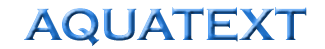Weir flow calculations

 Weir Type Calculation Horizontal, sharp edged weirs (Bazins formula) Q = 0.66 x cB x (2g)0.66 x H1.5 where; Q = water flow rate, m3/sec B = width of the weir, metres* c = discharge coefficient, average 0.62 g = gravitational constant, 9.81 H = Height of the water over the weir, measured behind the weir edge, m  * Note’; if the weir is narrower than the channel feeding it, shorten B by 0.2xH Broad crested (flat topped) weirs Q = 0.35 x B x (2g)0.5 x H1.5 where; Q = water flow rate, m3/sec B = width of the weir, metres g = gravitational constant, 9.81 H = h x (V2)/2g where, h = height of the water over the weir at the discharge edge of the weir, m V = velocity of the stream across the weir crest, m/sec V notch, sharp edged weirs Q = 0.266 x cB x (2g)0.5 x H1.5 where; Q = water flow rate, m3/sec B = width of the weir at the flowing rate c = discharge coefficient, average 0.62 g = gravitational constant, 9.81 H = Height of the water over the weir, measured behind the weir edge, m